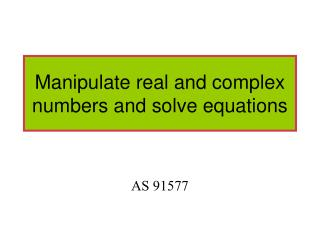Download PresentationManipulate real and complex numbers and solve equations

Manipulate real and complex numbers and solve equations - PowerPoint PPT Presentation

Manipulate real and complex numbers and solve equations. AS 91577. Worksheet 1. Quadratics. General formula:. General solution:. Example 1. Equation cannot be factorised. Using quadratic formula. We use the substitution. A complex number. The equation has 2 complex solutions. Imaginary.I am the owner, or an agent authorized to act on behalf of the owner, of the copyrighted work described.
Download PresentationManipulate real and complex numbers and solve equations

Download Policy: Content on the Website is provided to you AS IS for your information and personal use and may not be sold / licensed / shared on other websites without getting consent from its author.While downloading, if for some reason you are not able to download a presentation, the publisher may have deleted the file from their server.

- - - - - - - - - - - - - - - - - - - - - - - - - - E N D - - - - - - - - - - - - - - - - - - - - - - - - - -
Presentation Transcript
1. Worksheet 1

2. Quadratics General formula: General solution:

3. Example 1 Equation cannot be factorised.

4. Using quadratic formula We use the substitution A complex number

5. The equation has 2 complex solutions Imaginary Real

6. Equation has 2 complex solutions.

7. Example 2

8. Example 2

9. Example 2

10. Adding complex numbers Subtracting complex numbers

11. Example

12. Example

13. (x + yi)(u + vi) = (xu – yv) + (xv + yu)i. Multiplying Complex Numbers

14. Example

15. Example

16. Example 2

17. Conjugate If The conjugate of z is If The conjugate of z is

18. Dividing Complex Numbers

19. Example

20. Example

21. Example

22. Solving by matching terms Match real and imaginary Real Imaginary

23. Solving polynomials Quadratics: 2 solutions 2 real roots 2 complex roots

24. If coefficients are all real, imaginary roots are in conjugate pairs

25. If coefficients are all real, imaginary roots are in conjugate pairs

26. Cubic Cubics: 3 solutions 3 real roots 1 real and 2 complex roots

27. Quartic Quartic: 4 solutions 2 real and 2 imaginary roots 4 real roots 4 imaginary roots

28. Solving a cubic This cubic must have at least 1 real solutions Form the quadratic. Solve the quadratic for the other solutions x = 1, -1 - i, 1 + i

29. Finding other solutions when you are given one solution. Because coefficients are real, roots come in conjugate pairs so Form the quadratic i.e. Form the cubic:

30. Argand Diagram

31. Just mark the spot with a cross

32. z = i z = -1 z =1 z = -i

33. Reciprocal of z Conjugate

34. Rectangular to polar form Using Pythagoras Modulus is the length Argument is the angle Check the quadrant of the complex number

35. Modulus is the length

36. Example 1 Rectangular form Polar form

37. Example 2

38. Example 3

39. Converting from polar to rectangular

40. Multiplying numbers in polar form Example 1

41. Multiplying numbers in polar form Example 2 Take out multiples of